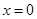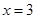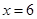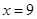# CLASS CHAPTER-16 PLAYING WITH NUMBERS

### Exercise 16.1

Find the values of the letters in each of the following and give reasons for the steps involved.

Question 1.

Solution :

On putting A = 1, 2, 3, 4, 5, 6, 7 and so on and we get, 7 + 5 = 12 in which ones place is 2.

∴ A = 7

And putting 2 and carry over 1, we get

B = 6

Hence A = 7 and B = 6

Question 2.

Solution :

On putting A = 1, 2, 3, 4, 5, 6, 7 and so on and we get,8 + 5 = 13 in which ones place is 3.

∴ A = 5

And putting 3 and carry over 1, we get

B = 4 and C = 1

Hence A = 5, B = 4 and C = 1

Question 3.

Solution :

On putting A = 1, 2, 3, 4, 5, 6, 7 and so on and we get, A x A = 6 x 6 = 36 in which ones place is 6.

∴ A = 6

Hence A = 6

Question 4.

Solution :

Here, we observe that B = 5

so that 7 + 5 = 12.

Putting 2 at ones place and carry over 1 and A = 2, we get

2 + 3 + 1 = 6

Hence A = 2 and B = 5

Question 5.

Solution :

Here on putting B = 0,

we get 0 × 3 = 0.

And A = 5, then 5 × 3 = 15

⇒ A = 5 and C = 1

Hence A = 5, B = 0 and C = 1

Question 6.

Solution :

On putting B = 0, we get 0 , 5 = 0 and A = 5, then 5×5 = 25

⇒ A = 5, C = 2

Hence A = 5, B = 0 and C = 2

Question 7.

Solution :

Here product of B and 6 must be same as ones place digit as B.

6 ×1 = 6, 6 ×2 = 12, 6 ×3 = 18,

6 ×4 = 24

On putting B = 4, we get the ones digit 4 and remaining two B’s value should be 44.

∴ For 6 × 7 = 42 + 2 = 44

Hence A = 7 and B = 4

Question 8.

Solution :

On putting B = 9, we get 9 + 1 = 10

Putting 0 at ones place and carry over 1, we get

For A = 7 ⇒ 7 + 1 + 1 = 9

Hence A = 7 and B = 9

Question 9.

Solution :

On putting B = 7,

⇒ 7 + 1 = 8

Now A = 4, then 4 + 7 = 11

Putting 1 at tens place and carry over 1, we get

2 + 4 + 1 = 7

Hence A = 4 and B = 7

Question 10.

Solution :

Putting A = 8 and B = 1, we get

8 + 1 = 9

Now again we add 2 + 8 = 10

Tens place digit is ‘0’ and carry over 1.

Now 1 + 6 + 1 = 8 = A

Hence A = 8 and B = 1

### NCERT Solutions for Class 8 Maths Exercise 16.1

Question 1.

If 21y5 is a multiple of 9, where y is a digit, what is the value of y?

Solution :

Since 21y5 is a multiple of 9.

Therefore according to the divisibility rule of 9, the sum of all the digits should be a multiple of 9.

∴ 2 + 1 y + 5 = 8 +y

⇒ 8 + y = 9

⇒ y = 1

Since 21y5 is a multiple of 9.

Question 2.

If 31z

Solution :

Since 31z5 is a multiple of 9.

Therefore according to the divisibility rule of 9, the sum of all the digits should be a multiple of 9.

∴ 3 + 1 + z + 5 = 9 + z

⇒ 9 + z = 9

⇒  z = 0

If  3 + 1 + z + 5 = 9 + z

⇒ 9 + z = 18

⇒ z = 9

Hence 0 and 9 are two possible answers.

Question 3.

If 24x is a multiple of 3, where x is a digit, what is the value of x?

(Since 24x is a multiple of 3, its sum of digits 6 + x is a multiple of 3; so 6 + x is one of these numbers: 0, 3, 6, 9, 12, 15, 18, … .But since x is a digit, it can only be that
6 + x = 6 or 9 or 12 or 15. Therefore, x = 0 or 3 or 6 or 9. Thus, x can have any of (four different values.)

Solution :

Since 24x is a multiple of 3.

Therefore according to the divisibility rule of 3, the sum of all the digits should be a multiple of 3.

∴ 2 + 4 + x = 6 + x

Since x is a digit.

⇒6 + x = 6 ⇒⇒ 6 + x = 9 ⇒⇒ 6 + x = 12  ⇒⇒ 6 + x = 15  ⇒Thus, x can have any of four different values.

Question 4.

If 31z5 is a multiple of 3, where z is a digit, what might be the values of z?

Solution :

Since 31z5 is a multiple of 3.

Therefore according to the divisibility rule of 3, the sum of all the digits should be a multiple of 3.

Since z is a digit.

∴ 3 + 1 + z + 5 = 9 +z

⇒ 9 +z = 9 ⇒ z = 0

If  3 + 1 + z + 5  = 9 + z

⇒ 9 + z = 12  ⇒ z = 3

If  3 + 1 + z + 5  = 9 + z

⇒ 9 + z = 15 ⇒ z = 6

If  3 + 1 + z + 5  = 9 + z

⇒ 9 + z = 15 ⇒ z = 9

Hence 0, 3, 6 and 9 are four possible answers.

Get 30% off your first purchase!

X
error: Content is protected !!
Scroll to Top